The Australian Journal of Mathematical Analysis and Applications

 Home News Editors Volumes RGMIA Subscriptions Authors Contact

ISSN 1449-5910

Total of 54 results found in site

8: Paper Source PDF document

Paper's Title:

A Comparison Between Two Different Stochastic Epidemic Models with Respect to the Entropy

Author(s):

Department of Mathematics,
University of Sussex,
Brighton BN1 9QH,
UK.
E-mail: f.fatehi@sussex.ac.uk
URL: http://www.sussex.ac.uk/profiles/361251

Department of Pure Mathematics, Faculty of Mathematics and Computer,
Shahid Bahonar University of Kerman,
Kerman 76169-14111,
Iran.

Abstract:

In this paper at first a brief history of mathematical models is presented with the aim to clarify the reliability of stochastic models over deterministic models. Next, the necessary background about random variables and stochastic processes, especially Markov chains and the entropy are introduced. After that, entropy of SIR stochastic models is computed and it is proven that an epidemic will disappear after a long time. Entropy of a stochastic mathematical model determines the average uncertainty about the outcome of that random experiment. At the end, we introduce a chain binomial epidemic model and compute its entropy, which is then compared with the DTMC SIR epidemic model to show which one is nearer to reality.

7: Paper Source PDF document

Paper's Title:

Solving Two Point Boundary Value Problems by Modified Sumudu Transform Homotopy Perturbation Method

Author(s):

Asem AL Nemrat and Zarita Zainuddin

School of Mathematical Sciences,
Universiti Sains Malaysia,
11800 Penang,
Malaysia.
E-mail: alnemrata@yahoo.com
zarita@usm.my

Abstract:

This paper considers a combined form of the Sumudu transform with the modified homotopy perturbation method (MHPM) to find approximate and analytical solutions for nonlinear two point boundary value problems. This method is called the modified Sumudu transform homotopy perturbation method (MSTHPM). The suggested technique avoids the round-off errors and finds the solution without any restrictive assumptions or discretization. We will introduce an appropriate initial approximation and furthermore, the residual error will be canceled in some points of the interval (RECP). Only a first order approximation of MSTHPM will be required, as compared to STHPM, which needs more iterations for the same cases of study. After comparing figures between approximate, MSTHPM, STHPM and numerical solutions, it is found through the solutions we have obtained that they are highly accurate, indicating that the MSTHPM is very effective, simple and can be used to solve other types of nonlinear boundary value problems (BVPs).

5: Paper Source PDF document

Paper's Title:

p-valent Meromorphic Functions Involving Hypergeometric and Koebe Functions by Using Differential Operator

Author(s):

S. Najafzadeh, S. R. Kulkarni and G. Murugusundaramoorthy

Department of Mathematics,
Fergusson College, Pune University,
Pune - 411004,
India.
kulkarni_ferg@yahoo.com

School of Science and Humanities,
Vellore Institute of Technology, Deemed University,
Vellore - 632014,
India.
gmsmoorthy@yahoo.com

Abstract:

New classes of multivalent meromorphic functions involving hypergeometric and Koebe functions are introduced,we find some properties of these classes e.g. distortion bounds, radii of starlikeness and convexity, extreme points, Hadamard product and verify effect of some integral operator on members of these classes.

5: Paper Source PDF document

Paper's Title:

On Closed Range C*-modular Operators

Author(s):

Department of Mathematics,
Faculty of Mathematics and Statistics Sciences,
University of Birjand, Birjand,
Iran.

Abstract:

In this paper, for the class of the modular operators on Hilbert C*-modules, we give the conditions to closedness of their ranges. Also, the equivalence conditions for the closedness of the range of the modular projections on Hilbert C*-modules are discussed. Moreover, the mixed reverse order law for the Moore-Penrose invertible modular operators are given.

5: Paper Source PDF document

Paper's Title:

Applications of Von Neumann Algebras to Rigidity Problems of (2-Step) Riemannian (Nil-)Manifolds

Author(s):

DFouman Faculty of Engineering,
College of Engineering, University of Tehran,
Iran.

Department of Mathematical Sciences,
Sharif University of Technology,
Iran
E-mail: fanai@sharif.edu

Abstract:

In this paper, basic notions of von Neumann algebra and its direct analogues in the realm of groupoids and measure spaces have been considered. By recovering the action of a locally compact Lie group from a crossed product of a von Neumann algebra, other proof of one of a geometric propositions of O'Neil and an extension of it has been proposed. Also, using the advanced exploration of nilmanifolds in measure spaces and their corresponding automorphisms (Lie algebraic derivations) a different proof of an analytic theorem of Gordon and Mao has been attained. These two propositions are of the most important ones for rigidity problems of Riemannian manifolds especially 2-step nilmanifolds.

4: Paper Source PDF document

Paper's Title:

Strong Convergence Theorem for a Common Fixed Point of an Infinite Family of J-nonexpansive Maps with Applications

Author(s):

Charlse Ejike Chidume, Otubo Emmanuel Ezzaka and Chinedu Godwin Ezea

African University of Science and Technology,
Abuja,
Nigeria.
E-mail: cchidume@aust.edu.ng

Ebonyi State University,
Abakaliki,
Nigeria.
E-mail: mrzzaka@yahoo.com

Nnamdi Azikiwe University,
Awka,
Nigeria.
E-mail: chinedu.ezea@gmail.com

Abstract:

Let E be a uniformly convex and uniformly smooth real Banach space with dual space E*. Let {Ti}i=1 be a family of J-nonexpansive maps, where, for each i,~Ti maps E to 2E*. A new class of maps, J-nonexpansive maps from E to E*, an analogue of nonexpansive self maps of E, is introduced. Assuming that the set of common J-fixed points of {Ti}i=1 is nonempty, an iterative scheme is constructed and proved to converge strongly to a point x* in n=1FJTi. This result is then applied, in the case that E is a real Hilbert space to obtain a strong convergence theorem for approximation of a common fixed point for an infinite family of nonexpansive maps, assuming existences. The theorem obtained is compared with some important results in the literature. Finally, the technique of proof is also of independent interest.

4: Paper Source PDF document

Paper's Title:

Strong Convergence Theorems for a Common Zero of an Infinite Family of Gamma-Inverse Strongly Monotone Maps with Applications

Author(s):

Charles Ejike Chidume, Ogonnaya Michael Romanus, and Ukamaka Victoria Nnyaba

African University of Science and Technology, Abuja,
Nigeria.
E-mail: cchidume@aust.edu.ng
E-mail: romanusogonnaya@gmail.com
E-mail: nnyabavictoriau@gmail.com

Abstract:

Let E be a uniformly convex and uniformly smooth real Banach space with dual space E* and let Ak:EE*, k=1, 2, 3 , ...
be a family of inverse strongly monotone maps such that k=1 Ak-1(0)≠∅.
A new iterative algorithm is constructed and proved to converge strongly to a common zero of the family.
As a consequence of this result, a strong convergence theorem for approximating a common J-fixed point for an infinite family of
gamma-strictly J-pseudocontractive maps is proved. These results are new and improve recent results obtained for these classes of nonlinear maps.
Furthermore, the technique of proof is of independent interest.

3: Paper Source PDF document

Paper's Title:

Certain Inequalities for P_Valent Meromorphic Functions with Alternating Coefficients Based on Integral Operator

Author(s):

Department of Mathematics, Faculty of Science
Urmia University, Urmia,
Iran
sa40shams@yahoo.com

Department of Mathematics, Faculty of Science
Maragheh University, Maragheh,
Iran

Abstract:

In this paper we introduce the class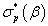of functions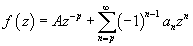regular and  multivalent in the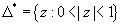and satisfying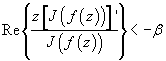where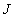is a linear operator.
Coefficient inequalities, distortion bounds, weighted mean and arithmetic mean of functions for this class have been obtained.

3: Paper Source PDF document

Paper's Title:

On ε-simultaneous Approximation in Quotient Spaces

Author(s):

H. Alizadeh, Sh. Rezapour, S. M. Vaezpour

Islamic University, Science and Research Branch, Tehran,
Iran

Department of Mathematics, Azarbaidjan
University of Tarbiat Moallem, Tabriz,
Iran

Department of Mathematics, Amirkabir
University of Technology, Tehran,
Iran

sh.rezapour@azaruniv.edu
vaez@aut.ac.ir

URL:http://www.azaruniv.edu/~rezapour
URL:http://math-cs.aut.ac.ir/vaezpour

Abstract:

The purpose of this paper is to develop a theory of best simultaneous approximation to ε-simultaneous approximation. We shall introduce the concept of ε-simultaneous pseudo Chebyshev, ε-simultaneous quasi Chebyshev and ε-simultaneous weakly Chebyshev subspaces of a Banach space. Then, it will be determined under what conditions these subspaces are transmitted to and from quotient spaces.

2: Paper Source PDF document

Paper's Title:

Presentation a mathematical model for bone metastases control by using tamoxifen

Author(s):

Maryam Nikbakht, Alireza Fakharzadeh Jahromi and Aghileh Heydari

Department of Mathematics,
Payame Noor University,
P.O.Box 19395-3697, Tehran,
Iran.
.E-mail: maryam_nikbakht@pnu.ac.ir

Department of Mathematics,
Faculty of Basic Science,
Shiraz University of Technology.

Department of Mathematics,
Payame Noor University,
P.O.Box 19395-3697, Tehran,
Iran.
E-mail: a-heidari@pnu.ac.ir

Abstract:

Bone is a common site for metastases (secondary tumor) because of breast and prostate cancer. According to our evaluations the mathematical aspect of the effect of drug in bone metastases has not been studied yet. Hence, this paper suggested a new mathematical model for bone metastases control by using tamoxifen. The proposed model is a system of nonlinear partial differential equations. In this paper our purpose is to present a control model for bone metastases. At end by some numerical simulations, the proposed model is examined by using physician.

2: Paper Source PDF document

Paper's Title:

Credibility Based Fuzzy Entropy Measure

Author(s):

G. Yari, M. Rahimi, B. Moomivand and P. Kumar

Department of Mathematics,
Iran University of Science and Technology,
Tehran,
Iran.
E-mail: Yari@iust.ac.ir
E-mail: Mt_Rahimi@iust.ac.ir
URL: http://www.iust.ac.ir/find.php?item=30.11101.20484.en
URL: http://webpages.iust.ac.ir/mt_rahimi/en.html

Qarzol-hasaneh
Mehr Iran Bank, Tehran,
Iran.
E-mail: B.moomivand@qmb.ir

Department of Mathematics and Statistics,
University of Northern British Columbia,
Prince George, BC,
E-mail: Pranesh.Kumar@unbc.ca

Abstract:

Fuzzy entropy is the entropy of a fuzzy variable, loosely representing the information of uncertainty. This paper, first examines both previous membership and credibility based entropy measures in fuzzy environment, and then suggests an extended credibility based measure which satisfies mostly in Du Luca and Termini axioms. Furthermore, using credibility and the proposed measure, the relative entropy is defined to measure uncertainty between fuzzy numbers. Finally we provide some properties of this Credibility based fuzzy entropy measure and to clarify, give some examples.

2: Paper Source PDF document

Paper's Title:

Robust Error Analysis of Solutions to Nonlinear Volterra Integral Equation in Lp Spaces

Author(s):

Department of Mathematics, Faculty of Mathematics,
University of Sistan and Baluchestan, P.O. Box 98135-674, Zahedan,
Iran.
E-mail: h.baghani@gmail.com

Department of Mathematics, Faculty of Basic Sciences,
Birjand University of Technology, Birjand,
Iran.
E-mail: j.farrokhi@birjandut.ac.ir

Department of Mathematics and Computer Sciences,
Hakim Sabzevari University, P.O. Box 397, Sabzevar,
Iran.
E-mail: o.baghani@gmail.com

Abstract:

In this paper, we propose a novel strategy for proving an important inequality for a contraction integral equations. The obtained inequality allows us to express our iterative algorithm using a "for loop" rather than a "while loop". The main tool used in this paper is the fixed point theorem in the Lebesgue space. Also, a numerical example shows the efficiency and the accuracy of the proposed scheme.

1: Paper Source PDF document

Paper's Title:

Approximation of an AQCQ-Functional Equation and its Applications

Author(s):

Choonkil Park and Jung Rye Lee

Department of Mathematics,
Research Institute for Natural Sciences,
Hanyang University, Seoul 133-791,
Korea;

Department of Mathematics,
Daejin University,
Kyeonggi 487-711,
Korea

baak@hanyang.ac.kr
jrlee@daejin.ac.kr

Abstract:

This paper is a survey on the generalized Hyers-Ulam stability of an AQCQ-functional equation in several spaces. Its content is divided into the following sections:

1. Introduction and preliminaries.

2. Generalized Hyers-Ulam stability of an AQCQ-functional equation in Banach spaces: direct method.

3. Generalized Hyers-Ulam stability of an AQCQ-functional equation in Banach spaces: fixed point method.

4. Generalized Hyers-Ulam stability of an AQCQ-functional equation in random Banach spaces: direct method.

5. Generalized Hyers-Ulam stability of an AQCQ-functional equation in random Banach spaces: fixed point method.

6. Generalized Hyers-Ulam stability of an AQCQ-functional equation in non-Archi-medean Banach spaces: direct method.

7. Generalized Hyers-Ulam stability of an AQCQ-functional equation in non-Archi-medean Banach spaces: fixed point method.

1: Paper Source PDF document

Paper's Title:

Solving Fractional Transport Equation via Walsh Function

Author(s):

L. M. F. N., Mathematics Department,
University of Setif,
Algeria
abdelouahak@yahoo.fr

Abstract:

In this paper we give a complete proof of A method for the solution of fractional transport equation in three-dimensional case by using Walsh function is presented. The main characteristic of this technique is that it reduces these problems to those of solving a system of algebraic equations, thus greatly simplifying the problem.

1: Paper Source PDF document

Paper's Title:

Euler Series Solutions for Linear Integral Equations

Author(s):

Department of Mathematics,
University of Msila 28000,
ALGERIA.
E-mail: dilmiistapha@yahoo.fr

Abstract:

In this work, we seek the approximate solution of linear integral equations by truncation Euler series approximation. After substituting the Euler expansions for the given functions of the equation and the unknown one, the equation reduces to a linear system, the solution of this latter gives the Euler coefficients and thereafter the solution of the equation. The convergence and the error analysis of this method are discussed. Finally, we compare our numerical results by others.

1: Paper Source PDF document

Paper's Title:

Fractional exp(-φ(ξ))- Expansion Method and its Application to Space--Time Nonlinear Fractional Equations

Author(s):

A. A. Moussa and L. A. Alhakim

Department of Management Information System and Production Management,
College of Business and Economics, Qassim University,
P.O. BOX 6666, Buraidah: 51452,
Saudi Arabia.
E-mail: Alaamath81@gmail.com

Department of Management Information System and Production Management,
College of Business and Economics, Qassim University,
P.O. BOX 6666, Buraidah: 51452,
Saudi Arabia.
E-mail: Lama2736@gmail.com